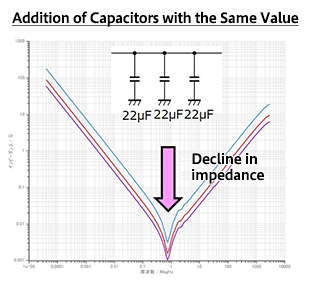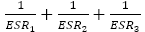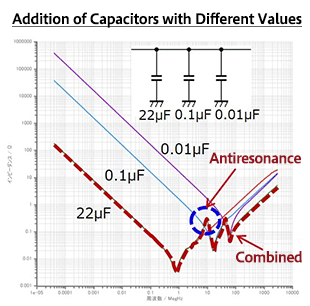Technical Information Site of Power Supply Design

2018.10.25 Switching Noise - EMC

# Effective Use of Decoupling (Bypass) Capacitors Point 1

Dealing with Noise Using Capacitors

In the article before last and in the last article, we explained the frequency characteristics of capacitors and how they are used in noise reduction. In this and the next two articles, we will explain the effective use of decoupling capacitors.

Effective Use of Decoupling Capacitors

Broadly speaking, there are two important points to remember for the effective use of decoupling capacitors. Apart from these, there are also some matters to be noted. In this article, among these three topics for discussion, we shall focus on Point 1 below.

• Effective Use of Decoupling (Bypass) Capacitors Point 1
• Point 2: Reducing the capacitor ESL (equivalent series inductance)
• Other matters to be noted

Point 1: Use of Multiple Decoupling Capacitors

One effective method of decoupling capacitor use involves use not of a single capacitor, but of multiple capacitors for decoupling. When using multiple capacitors, the effect is different when using capacitors with the same capacitance and when using a combination of capacitors with different capacitance values.

・When using multiple capacitors with the same capacitance value

The graph on the right indicates the frequency characteristics for cases in which a single 22 μF capacitor is used (blue), in which another such capacitor is added (red), and in which still another is added, to use three capacitors (purple).

As the graph indicates, when capacitors with the same capacitance value are added, the impedance is shifted downward, that is to say, the impedance is lowered, over the entire frequency range.

This can be understood when one considers the capacitive characteristic up to the resonance point when capacitors with the same value are connected in parallel, the fact that the resonance-point impedance depends on the ESR (equivalent series resistance), and the inductive characteristic due to the ESL (equivalent series inductance) beyond the resonance point.

Capacitances connected in parallel are additive, and so when three such capacitors are connected the capacitance is 66 μF, and the impedance in the capacitive region decreases.The ESRs of three capacitors are connected in parallel, and so the impedance at the resonance point is, and if the ESR values of all the capacitors are assumed to be the same, then the ESR is reduced to 1/3 and the impedance is also reduced.

The ESLs of the capacitors in the inductive region beyond the resonance point are also in parallel, so thatresults, and if the ESL values of the three capacitors are all the same, the ESL is reduced to 1/3 and the impedance is also reduced.

In this way, by using multiple capacitors with the same value, the impedance can be lowered over the entire frequency range, and so noise can be further attenuated.

・When using multiple capacitors with different capacitance values

This is the frequency characteristic for a case in which capacitors with values of 22 μF, 0.1 μF, and 0.01 μF are added in parallel.

By adding a capacitor with a small capacitance, the impedance at high frequencies can be lowered. The frequency characteristics of 0.1 μF and 0.01 μF capacitors are combined with the characteristic of a lone 22 μF capacitor, resulting in the characteristic shown (broken red line).

What requires attention here is the fact that an antiresonance may occur depending on the frequency, so that impedance is increased rather than decreased, and the EMI is worsened. An antiresonance occurs at the point at which the capacitive characteristic and the inductive characteristic intersect.In general, the value of a capacitance to be added is selected according to the frequency of the noise to be reduced.

The frequency characteristic diagrams shown here are ideal examples, and parasitic components due to pattern wiring in the circuit board and the like have not been considered. When taking actual measures to counter noise, the effects of parasitic components must be included. In the next article, the second point mentioned above will be explained.

#### Key Points:

・There are two main points to consider for the effective use of decoupling capacitors: (1) Use of multiple capacitors, and (2) lowering capacitor ESL values.

・When using multiple capacitors, the effect differs depending on whether the capacitance values are all the same or are different.

This website uses cookies.

By continuing to browse this website without changing your web-browser cookie settings, you are agreeing to our use of cookies.